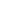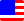Documents  >  Tutorial  >  Expressions
Expressions

An element that returns an instance

An expression is an element that returns an instance.

The minimum expression in Rice is the following two.

Reference

Literal

These are expressions that return an instances by themselves.

The reference returns the instance associated with the name, and the literal returns the instance that represents the constant.

You can generate a new expression by combining operators with the instances returned by the expression. Moreover, you can repeat such process as many as necessary.

As a result, an instance will be returned. This is the result of the whole expression.

For example.

int i = 5; // ♦1

int j = 5; // ♦1

int k = i + j + 10; // ♦2

♦1 defines a variable by the instance returned by the literal. As a result, these variables refer to an instance of the int class. Its value is 5.

♦2 applies the addition operator to the instances referred to by i and j. As a result, new instance of the int class are returned. Its value is 10.

The addition operator applies to the instance and the instance returned by the literal. And, new instance of the int class is returned again. Its value is 20. The variable k is defined in this instance.